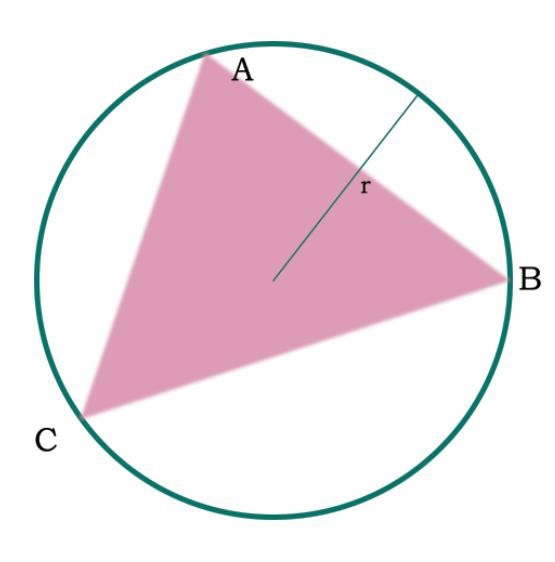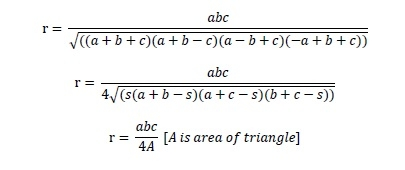# Area of the circumcircle of any triangles with sides given in C++

Here we will see how to get the area of the circumcircle of any triangle whose sides are given. Here the side AB is a, BC is b and CA is c, the radius is ‘r’.The radius r is same as −## Example

#include <iostream>
#include <cmath>
using namespace std;
float area(float a, float b, float c) {
if (a < 0 || b < 0 || c < 0) //if values are is negative it is invalid
return -1;
float s = (a + b + c) /2;
float triangle = sqrt(s * (s - a) * (s - b) * (s - c));
float area = 3.14159 * pow(((a*b*c) / triangle), 2);
return area;
}
int main() {
float a = 4, b = 5, c = 3;
cout << "Area : " << area(a, b, c);
}

## Output

Area : 314.159